### Home > A2C > Chapter Ch9 > Lesson 9.2.2 > Problem9-89

9-89.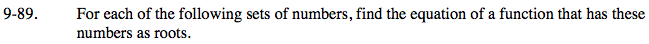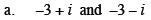If these are the roots, what are the factors?

y = [x − (−3 + i)] [x − (−3 − i)]

Mutiply the factors. You may want to use a generic rectangle. Simplify after multiplying.

$\textit{f}(\textit{x})=\textit{x}^2+6\textit{x}+10$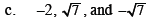What are the factors?

$\textit{y} =\left( \textit{x}-(-2) \right) \left( \textit{x}-\sqrt{7} \right) \left( \textit{x}+\sqrt{7} \right)$

Multiply the last two factors first.

$\textit{h}(\textit{x})=\textit{x}^3+2\textit{x}^2-7\textit{x}-14$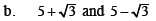Use the same process as part (a) above.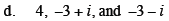Use the ideas from part (a) and part (c) for this problem. How does part (a) make this problem reasonable?